A block of mass m moving due east at speed v collides with and sticks to a block of mass 2m that is moving at the same speed vv but in a dir

Question

A block of mass m moving due east at speed v collides with and sticks to a block of mass 2m that is moving at the same speed vv but in a direction 45.0∘ north of east. Find the direction in which the two blocks move after the collision. Express your answer as an angle θ in degrees measured north of east.

in progress 0
6 months 2021-07-19T22:37:19+00:00 1 Answers 13 views 0

30.36°

Explanation:

By using linear momentum; linear momentum can be expressed by the relation: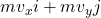where ;

m= mass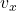= velocity of components in the x direction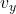= velocity of components in the y direction

If we consider the east as the positive x and north as positive y which is synonymous to what we usually have on a graph.

Then;

Initial momentum =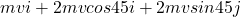=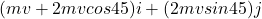However, the masses stick together after collision and move with a common velocity: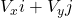∴ Final momentum =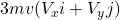=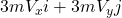From the foregoing ;

initial momentum = final momentum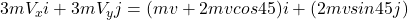So;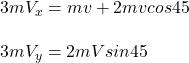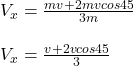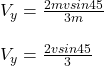Finally;

The required angle θ =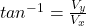θ =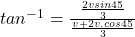θ =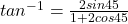θ = 30.36°